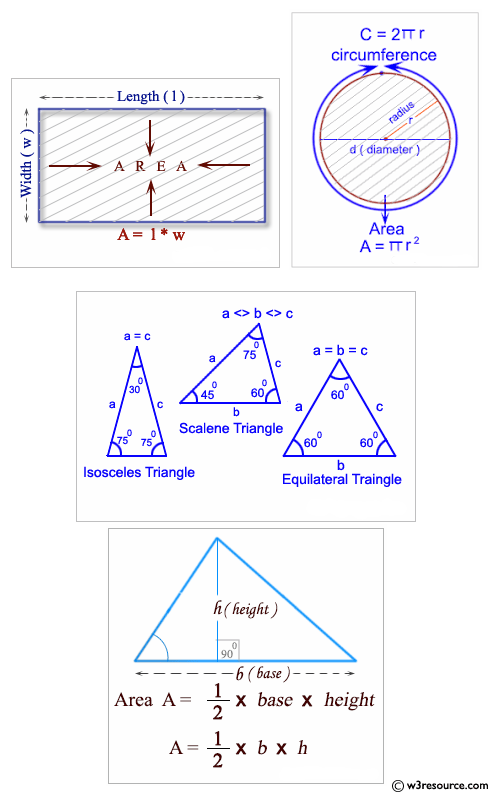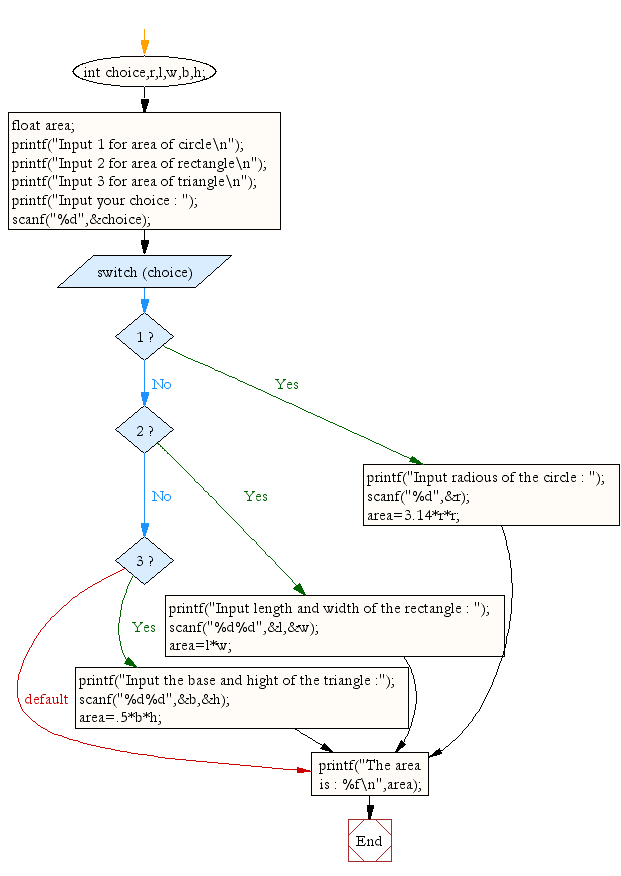﻿ C Program: Compute the area of the various geometrical shape# C Exercises: A menu-driven program to compute the area of the various geometrical shape

## C Conditional Statement: Exercise-25 with Solution

Write a program in C which is a Menu-Driven Program to compute the area of the various geometrical shape.

Pictorial Presentation:Sample Solution:

C Code:

``````#include <stdio.h>
void main ()
{
int choice,r,l,w,b,h;
float area;
printf("Input 1 for area of circle\n");
printf("Input 2 for area of rectangle\n");
printf("Input 3 for area of triangle\n");
scanf("%d",&choice);
switch(choice)
{
case 1:
printf("Input radious of the circle : ");
scanf("%d",&r);
area=3.14*r*r;
break;
case 2:
printf("Input length and width of the rectangle : ");
scanf("%d%d",&l,&w);
area=l*w;
break;
case 3:
printf("Input the base and hight of the triangle :");
scanf("%d%d",&b,&h);
area=.5*b*h;
break;
}
printf("The area is : %f\n",area);
}
```
```

Sample Output:

```Input 1 for area of circle
Input 2 for area of rectangle
Input 3 for area of triangle
Input radious of the circle : 5
The area is : 78.500000
```

Flowchart:C Programming Code Editor:

Improve this sample solution and post your code through Disqus.

What is the difficulty level of this exercise?

Test your Programming skills with w3resource's quiz.

﻿

## C Programming: Tips of the Day

Why do C and C++ compilers allow array lengths in function signatures when they're never enforced?

It is a quirk of the syntax for passing arrays to functions.

Actually it is not possible to pass an array in C. If you write syntax that looks like it should pass the array, what actually happens is that a pointer to the first element of the array is passed instead.

Since the pointer does not include any length information, the contents of your [] in the function formal parameter list are actually ignored.

Ref : https://bit.ly/3fhlvdH